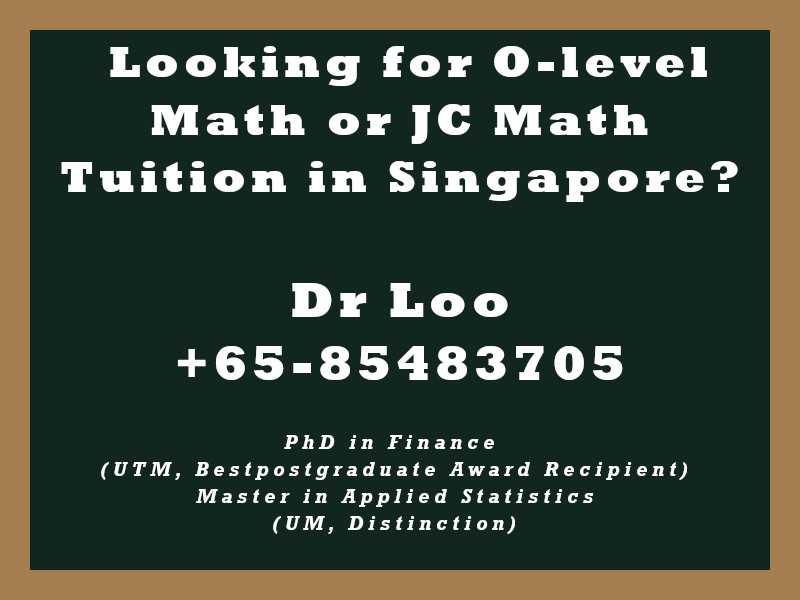+65-85483705

# Partial Fractions

A complex rational expression can be decomposed into simpler fractions. You would likely to come across this topic during the O-level a-math class.

Question

1. Express  (2x – 3)/(x2 – 3x + 2) as partial fractions.
2. Express  x-1/(x+2)(x-3) as partial fractions.

1. 1/(x-1) + 1/(x-2)
2. 1/(x+2) + 1/(x-3)

## About the Singapore Math Tutor - Dr Loo

I am a PhD holder with 9 years of teaching experience on teaching quantitative subjects at secondary school and university levels. My expertise are mathematics, statistics, econometrics, finance and machine learning.

Currently I do provide consultation and tuition for math tuition in Singapore. If you are looking for secondary math tutor in Singapore, JC H2 math tutor or statistics tutor in Singapore, please feel free to contact me at +65-85483705 (SMS/Whatsapp).

Among the Singapore math tuition that I provide, this includes Singapore secondary math tuition (E-math tuition & A-math tuition) and also JC Math Tuition (H1 Math Tuition & H2 Math Tuition). On the other hand, my statistics tuition in Singapore mostly focus on pre-university level until postgraduate level. For those who are looking for online tutoring service, the online O-level math tuition and online A-level math tuition are also available.